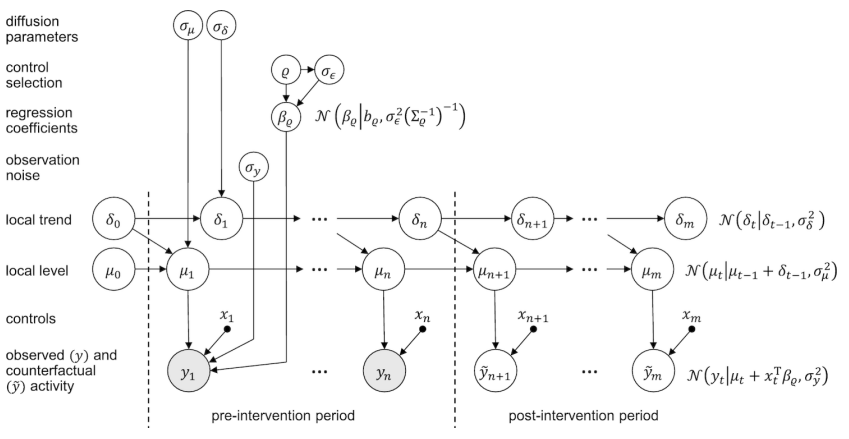# Causal Inference : An Introduction

Original article was published on Deep Learning on Medium# Causal Inference

The domain of causal inference is based on the simple principle of cause and effect, i.e., our actions directly cause an immediate effect. With causal inference, we can directly find out how changes in policy (or actions) create changes in real world outcomes. Let us familiarise ourselves with terminology used in the domain.

Unit : An individual sample in the data.

Variable : A characteristic of the unit of analysis in the dataset. For example, countries, people, weather, etc.

Population : Collected set of all units of analysis.

Outcome Variable : The particular variable that we want to affect.

Policy / Treatment Variable : The variable used to create changes (i.e. actions).

Intervention : Changing the value of the treatment variable keeping other variable values constant in order to study the effect of changes in the treatment variable.

Confounder : Variables that affect both the input and the outcome variables.

Counterfactual Outcome : The outcome that would have occurred had the unit been exposed to a different treatment (cannot be observed).

Unit Level Causal Effect : Causal effect on a single unit in the data due to a change in the value of a particular variable keeping all other variables constant.

Interaction Effects : This refers to the fact that other variables may also influence the value of a causal effect.

Heterogeneity : Refers to the concept that different units exhibit different causal effects on being subjected to the same treatment.

Given an distribution of input variables X and a distribution of outcome variables (effects) Y, causal calculus differentiates between two kinds of conditional distributions – the observational distribution P(y|x) and the interventional distribution P(y|do(x)). P(y|x) represents the distribution of Y given that we observe variable X takes value x whereas P(y|do(x)) describes the distribution of Y that would be observed by artificially forcing the variable X to take a value x, keeping the rest of the variables in accordance with the process that generated the data. Such interventional distributions can be obtained using randomized controlled trials (RCTs). However, it might not always be possible to carry out such experiments due to ethical or practical concerns. More about causal inference and its relation with do-calculus can be found here.

The Fundamental Problem of causal inference is that in the real world, each unit can be subjected to just one of the multiple treatments and only the outcome corresponding to that treatment can be observed. The effect of other treatments on the same unit cannot be directly observed and that is what we want to predict using causal inference techniques.

Note : Causation does not imply correlation. Correlation refers to how two variables are related whereas causation studies if one variable affects the others. Two variables might be varying in a correlated fashion but one of them might not be causing changes in the other.

## Frameworks for Causal Inference

Structural Equation Modelling (SEM). Structural equation modelling is a methodology for representing, estimating, and testing a network of relationships between variables (measured variables and latent constructs). The purpose of the model, in the most common form of SEM, is to account for variation and covariation of the measured variables.

Potential Outcomes Framework. Also known as the Rubin causal model (RCM), the potential outcomes framework is based on the idea of potential outcomes. For example, a person would have a particular income at age 40 if she had attended college, whereas she would have a different income at age 40 if she had not attended college. To measure the causal effect of going to college for this person, we need to compare the outcome for the same individual in both alternative futures. Since it is impossible to see both potential outcomes at once, one of the potential outcomes is always missing.